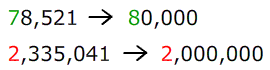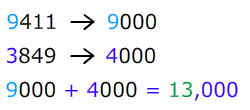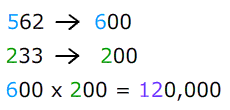# Front End Estimation

There are many ways to estimate an answer. One method is called "Front End Estimation."

The name comes from the way that you round. Instead of rounding each number to a given place value, we round whatever number is in the front.

Take a look at an example:

Use front end estimation to determine the sum of 78,251 and 2,335,041.We keep only one number in the front and all the others became zeros.Let's try another.

Use front end estimation to estimate the answer. 9411 + 3849

When we estimate, we must remember to ROUND FIRST!We can use front end estimation with other operations as well.

Example: Use the front end method to estimate the difference. 478 - 96Example: Use the front end method to estimate the product. 562 x 233Example: Use the front end method to estimate the quotient. 5875 ÷ 517Front end estimation is just one way of estimating an answer. You will need to round the number so that there is one digit in the front and all others become zeros. Then you are ready to complete any operations that may be asked.